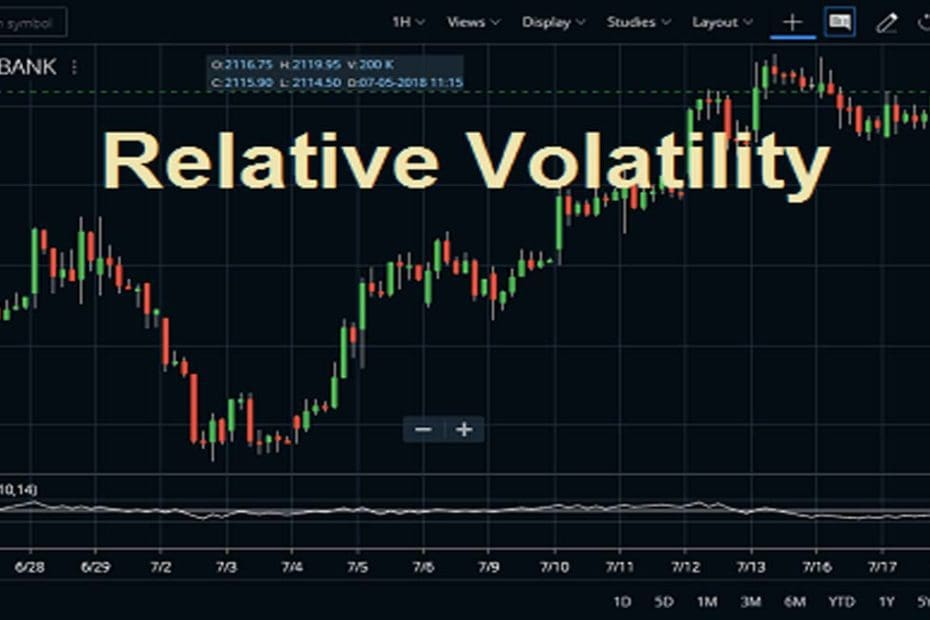Home » Blog » Trading » Technical Indicators » Relative Volatility Index (RVI) Indicator Strategy

# Relative Volatility Index (RVI) Indicator Strategy

• Technical IndicatorsDonald Dorsey developed the Relative Volatility Index (RVI) indicator. He actually understood that an indicator is not the holy grail of trading. The RVI indicator looks similar to the relative strength index. The basic difference is it measures the standard deviation (SD) of the high and low prices over a pre-defined range of periods.

## What is the Relative Volatility Index (RVI)?

The Relative Volatility Index is a confirming indicator, helps to determine the market volatility. Many get confused between the Relative Volailiy Index and the Relative Vigor Index. The relative volatility index indicator measures the relative volatility of the price compared to the previous period.

## Calculation

The RVI indicator is calculated in a similar way as the RSI but using the standard deviation or SD of high and low prices rather than the absolute change in the price.

Usum = sum (u)/n.
Dsum = sum (d)/n.

where n is the bar period selected by a user.

Usum is the averaged sum of STD for positive days. If the close price is above the previous close, then u = STD (n) otherwise, u = 0.
Dsum is the averaged sum of STD for negative days. If close is below the previous close, then d = STD (n) otherwise, d = 0.

## What is the Usage of the Relative Volatility Index (RVI) Indicator:

If traders want to know more about the indicator, then they can find it in the STUDIES section of Zerodha Kite. They can also use the Kite Mobile App to find out this RVI indicator. The Field is close and we can also set this. The STD Period is 10 and we can set it also. The Smoothing Period is 14 and we can change this value high and low. Check the image below to understand how to attach the RVI in HDFC Bank share price chart. You can attach this indicator on to any charts likes daily, weekly, monthly or intraday.

The next platform, I am going to describe is Upstox Pro. Here, I will show you how you can set up the RVI on your charts. First, open the chart and put the name of the indicator. After that, click on apply.

## How to Trade Successfully using the RVI Indicator?

When the Relative Volatility Index (RVI) is above 50 it indicates that the volatility is to the upside, and when it is below 50, it indicates that the direction of volatility is to the downside. Thus, when the Relative Volatility Index is above 50, it confirms a potential buy signal; and when it is below 50, it confirms a potential sell signal.

In some trading platforms, the RVI indicator is plotted around levels of 0 and 100 with the overbought and the oversold levels set to 70 and 30. This makes the indicator’s settings somewhat similar to the Stochastics oscillator or RSI (relative strength index) which also oscillates around the 70 and 30 levels.

The indicator was not designed as a standalone indicator, rather as a confirmation for trading signals. The RVI is most widely used in conjunction with moving average crossover signals.

One of the significant benefits of this method is that the trading system provides enough time to react. Volatility does not rise and disappear within a limited period of time. Hence, depending on the bullish or the bearish positioning of the moving averages, one can simply just keep adding to positions and lock in good profits.

The way the RV index behaves is nothing different from other oscillators that one would more widely use. For instance, the peaks and troughs formed in the relative volatility index are pretty much similar to what you would expect when using the relative strength index or the RSI indicator.

## Brief Description of Buy and Sell Signals of RVI:

• Buy if Relative Volatility Index (RVI) > 50.
• Sell if Relative Volatility Index (RVI) > 50.
• If a trader misses the first RVI buy signal buy when RVI > 60.
• If a trader misses the first RVI Sell signal sell when RVI < 40.
• When the RVI falls below 40 then close a long position.
• When the RVI rises above 60 then close a short position.

## Important Features of RVI

• The relative volatility index behaves in a similar way as most oscillators do. Hence, the relative volatility index can also signal multiple levels of volatility in the market.
• Once volatility rises, one can see that the indicator remains above the 50 level-handle. However, you can see a peak above the 80-level as a strong indicator of volatility in the stock markets.
• Over many years, most traders have designed their own volatility based on useful trading systems. One of the facts with the indicator is that it can be applied to any markets and multi time frame. As a result, this is one of the most popularly used indicators when it comes to day trading in the markets.
• On the other side, the relative volatility index is not much available in many trading or charting platforms. So, one either needs to build one or create a customized indicator that can measure volatility as the RVI does.
• So, trading with rising and falling volatility can provide traders an extra edge in the markets. This is possible by applying the relative volatility index which is a simple yet effective tool to ride the volatility within the trend.

## Conclusion

In conclusion, the relative volatility index is a simple but efficient market indicator that helps to measure the rise and fall in the volatility of an asset. Many traders use the particular index in the stock as well as the futures markets, but you can also apply this to the forex markets too.